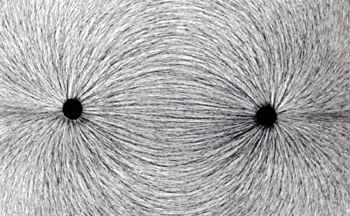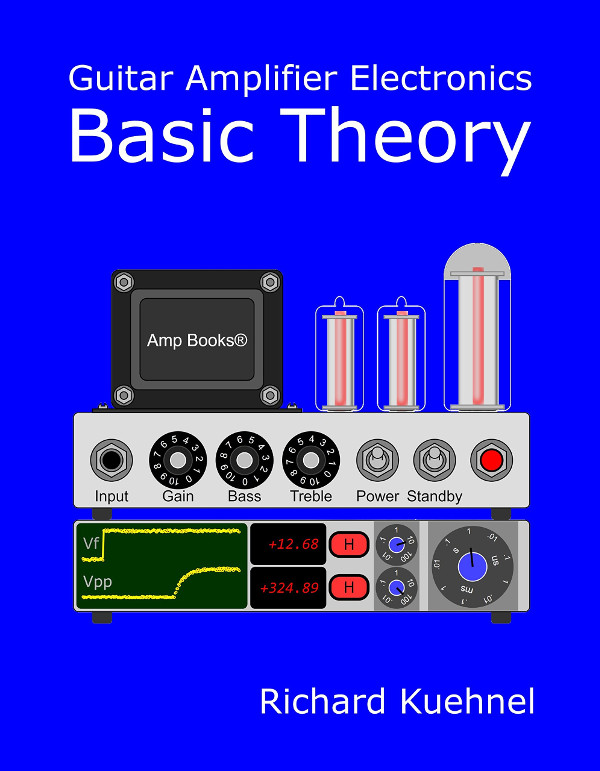I love to work on tube amps. It takes work to get them running, but once they work all that hard work really pays off. These are just some of the definitions of work that have no meaning to a physicist like Georg Simon Ohm. To him work is required to move an object against a force that opposes its motion. So lifting your Hiwatt DR103 from the floor to your work bench requires work, because you are moving the amp upwards against the force of gravity that opposes it. On the other hand, carrying a Twin Reverb around the block is no work at all, because the amp is moving horizontally - gravity does not oppose the movement in that direction. (So when the road crew complains just refer back to this tutorial and explain to them why there is no work involved.)

Moving the amp from your test bench to the floor takes work, in this case negative work because the direction of motion is helped along by the force of gravity. If all this sounds strange already, just add this concept to the mix: work is independent of time. It takes a specific amount of work to lift your DR103 to the bench. It doesn't matter whether you jerk it upwards in a fraction of a second or take 20 minutes to lift it over the same path. It is the same amount of work.

When the opposing force is measured in newtons and when the distance traveled against the opposing force is measured in meters, then the work, measured in joules, is the force times the distance:

W = Fd

Question: a 30 kilogram tube amp would be how many kilograms on the moon? Answer: 30 kilograms. It turns out that a kilogram is a unit of mass. The force that we think of when we try to lift that parallel push-pull boat anchor is the mass times the acceleration of gravity, which happens to be 9.8 meters per second squared. When we multiply the number of kilograms by 9.8, we get the force of earth's gravity measured in newtons.

#### Problem

The output transformer for your Traynor YGA-1 is mounted upside down and weighs 6.6 pounds. What is the force of gravity pulling it downward?

#### Solution

6.6 pounds is 6.6/2.2 = 3kg. The force of gravity in newtons is then

(3kg)(9.8m/s2) = 29NNew! Guitar Amplifier Electronics: Fender Deluxe - from TV front to narrow panel to brownface to blackface Reverb

#### Problem

Your vintage 6.6 pound output transformer is resting on the floor when you suddenly decide it would be perfect for your JTM45 re-issue. The top of your bench is 1.2 meters above the floor. How much work is required to pick up the transformer and place it on the bench? What if the transformer is sitting on the floor in the next room, which is 20 meters away?

#### Solution

We determined in the previous problem that the force of gravity on a 6.6 pound object is 29 newtons. The amount of work in joules is then

(29N)(1.2m) = 35J

Lateral movement is not opposed by gravity and the transformer in the next room travels the same vertical distance. It therefore takes the same amount of work, 35 joules.

#### Voltage

Opposite charges attract. Like charges repel. If we move a negative electron towards another electron we perform work because we are moving against an opposing force. Moving two electrons toward two other electrons requires more work because there is more opposing force. We often need a convenient way to describe how much work is required to move charge from one point to another. That concept is voltage.If moving a positive charge from point B to point A requires positive work, then point A is said to have a positive voltage with respect to B. The voltage in volts is equal to the work required in joules divided by the amount of charge in coulombs:

V = W/Q

Since W and Q can be positive or negative, it stands to reason that V can be positive or negative also.Guitar Amplifier Electronics: Basic Theory - master the basics of preamp, power amp, and power supply design.

#### Problem

It takes 1.6kJ (1600 joules) of energy to move 1x1020 electrons from the plate supply (Point A) through the plate load resistor to the plate (Point B) of your JTM45 preamp. (Notice that this is opposite to the actual electron flow shown here.) What is the voltage V across the resistor?

#### Solution

1x1020 electrons represent

(1x1020)(-1.6x10-19C) = -16C

of charge. Thus +1.6kJ of work is required to drive -16C from Point A to Point B. This means that it would take +1.6kJ of work to drive a positive charge of 16C from Point B to Point A. We therefore conclude that the voltage at A is positive with respect to B by an amount equal to

V = 1.6kJ / 16C = 100V

Thus there is a drop of 100 volts across the resistor from the plate supply to the plate.Fundamentals of Guitar Amplifier System Design - design your amp using a structured, professional methodology.

#### Power

Power is a measure of the amount of work or energy being expended over time. When measured over the period of one second, the number of joules of sonic energy created from an Ampeg SVT with six push-pull power tubes cranked full blast is going to be far more than from a Champ 5E1. By the same concept it takes no additional work to physically lift your Hiwatt quickly, but it takes more power because the work is performed over a shorter time period. When work is measured in joules and time is measured in seconds, then the power in watts is equal to the total work divided by the total time:

P = W/t

#### Problem

It takes you 2 seconds to steadily lift your 6.6 pound output transformer from the floor to the top of your bench, a total vertical distance of 1.2 meters. What is the average amount of power you are expending as you lift it?

#### Solution

We determined earlier that it takes 35 joules of work to lift the transformer. You expend that energy in 2 seconds so the average power, in watts, rendered upon the transformer by your arms and quadriceps is

P = 35J / 2s = 17W

#### The Relationship Between Voltage, Current, and Power

We have seen that the voltage between two points is an indication of the amount of work needed to move charge between the two points. Turning this concept around, work can be defined as the voltage between the two points times the charged that was moved between them:

W = VQ

We can use these concepts to derive a very important formula for guitar amplifiers. The relationship between voltage, current, and power is

P = W/t = (VQ)/t = V(Q/t) = VI

where the variable "I" is used to denote current. When I is in amps and V is in volts, then P is in watts.Guitar Amplifier Electronics: Circuit Simulation - know your design works by measuring performance at every point in the amplifier.

#### Problem

A steady current of 11mA flows through a Fender Bassman 5F6-A choke when no guitar signal is present. The choke is not a perfect inductor, because its internal windings have DC resistance that causes a 1.2 volt drop across it. How much power is the choke giving off in the form of heat?

#### Solution

Since the voltage across the choke and the current through it are steady (well, maybe a little AC ripple) the power given off as heat, measured in milliwatts, is

(1.2V)(11mA) = 13mW

#### Problem

The 47-ohm cathode resistor in the power amp of your Vox AC30 is rated at 10 watts maximum power. At idle the voltage across it is normally 10 volts. What would the average current through the resistor need to be to produce 10 watts of heat?New! A deep dive into the electronic design of the ultimate high-gain rig-of-death!From system design concepts to individual stage operation, an all-new examination of Bassman electronics.From "woody" to narrow panel to brownface to blackface Reverb, an in-depth exploration of Fender Deluxe circuitry.Master the basics of preamp, power amp, and power supply design.Design your amp using a structured, professional methodology.Know it works by measuring performance at every point in the amplifier.